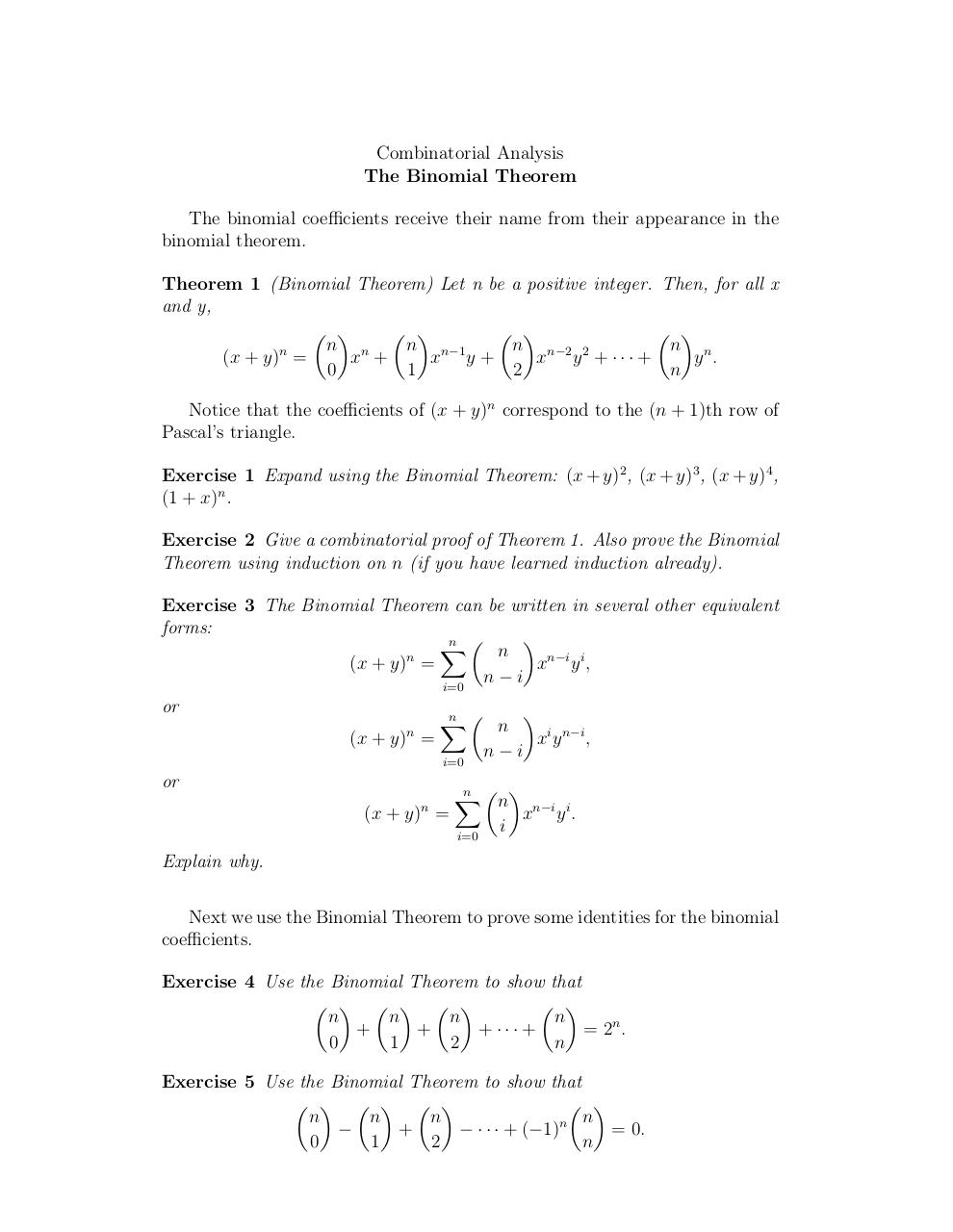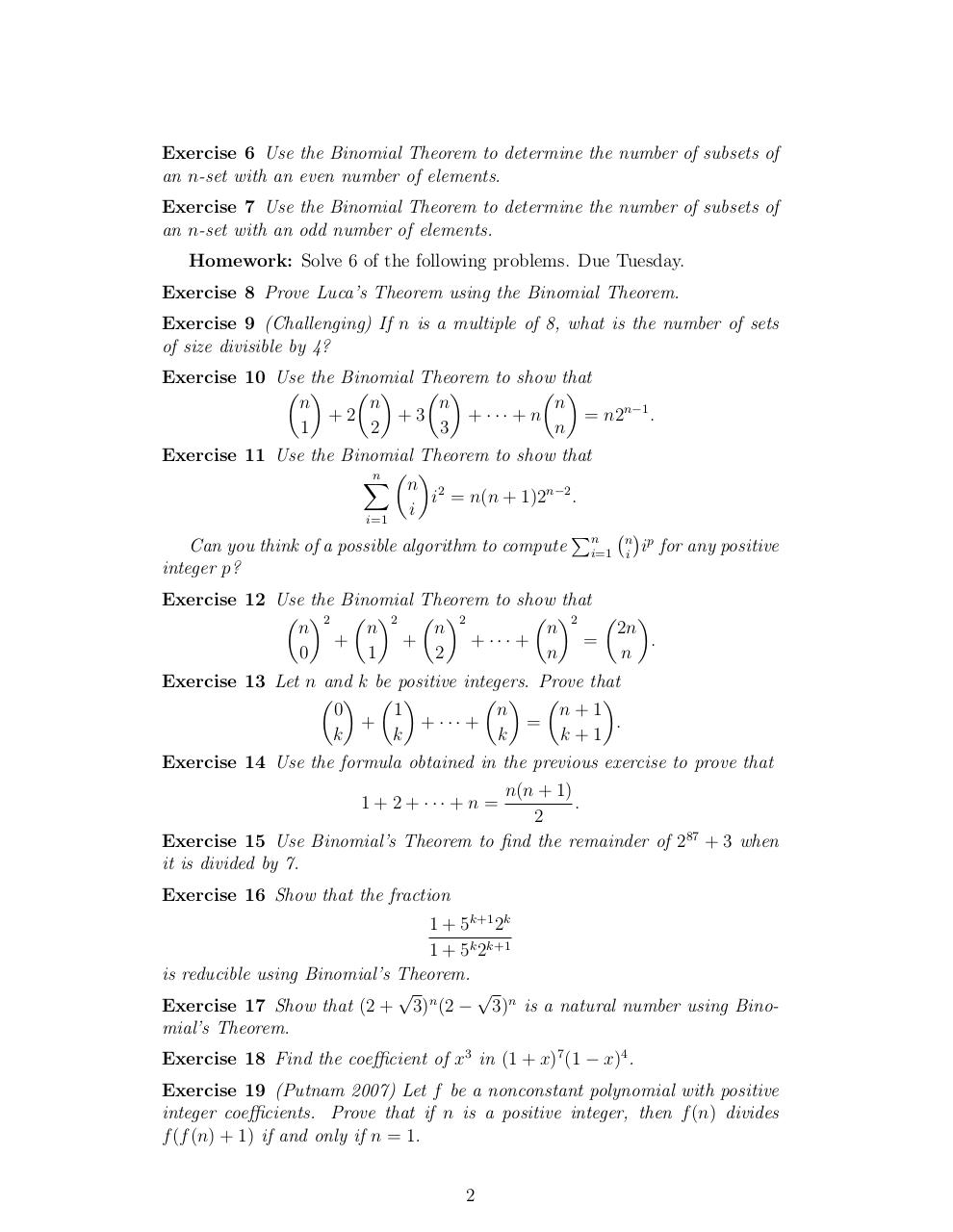# Day 13 Binomial Theorem .pdf

### File information

Original filename: Day 13- Binomial Theorem.pdf

This PDF 1.4 document has been generated by TeX / pdfTeX-1.40.3, and has been sent on pdf-archive.com on 24/01/2014 at 06:10, from IP address 169.231.x.x. The current document download page has been viewed 685 times.
File size: 98 KB (2 pages).
Privacy: public file

Day 13- Binomial Theorem.pdf (PDF, 98 KB)

### Document preview

Combinatorial Analysis
The Binomial Theorem
The binomial coefficients receive their name from their appearance in the
binomial theorem.
Theorem 1 (Binomial Theorem) Let n be a positive integer. Then, for all x
and y,
 
 
 
 
n n
n n−1
n n−2 2
n n
n
(x + y) =
x +
x y+
x y + ··· +
y .
0
1
2
n
Notice that the coefficients of (x + y)n correspond to the (n + 1)th row of
Pascal’s triangle.
Exercise 1 Expand using the Binomial Theorem: (x + y)2 , (x + y)3 , (x + y)4 ,
(1 + x)n .
Exercise 2 Give a combinatorial proof of Theorem 1. Also prove the Binomial
Theorem using induction on n (if you have learned induction already).
Exercise 3 The Binomial Theorem can be written in several other equivalent
forms:

n 
X
n
n
(x + y) =
xn−i y i ,
n

i
i=0
or


n 
X
n
(x + y) =
xi y n−i ,
n

i
i=0
n

or
n

(x + y) =

n  
X
n
i=0

i

xn−i y i .

Explain why.
Next we use the Binomial Theorem to prove some identities for the binomial
coefficients.
Exercise 4 Use the Binomial Theorem to show that
     
 
n
n
n
n
+
+
+ ··· +
= 2n .
0
1
2
n
Exercise 5 Use the Binomial Theorem to show that
     
 
n
n
n
n n

+
− · · · + (−1)
= 0.
0
1
2
n

Exercise 6 Use the Binomial Theorem to determine the number of subsets of
an n-set with an even number of elements.
Exercise 7 Use the Binomial Theorem to determine the number of subsets of
an n-set with an odd number of elements.
Homework: Solve 6 of the following problems. Due Tuesday.
Exercise 8 Prove Luca’s Theorem using the Binomial Theorem.
Exercise 9 (Challenging) If n is a multiple of 8, what is the number of sets
of size divisible by 4?
Exercise 10 Use the Binomial Theorem to show that
 
 
 
 
n
n
n
n
+2
+3
+ ··· + n
= n2n−1 .
1
2
3
n
Exercise 11 Use the Binomial Theorem to show that
n  
X
n 2
i = n(n + 1)2n−2 .
i
i=1
P
Can you think of a possible algorithm to compute ni=1
integer p?

n
i

p
i for any positive

Exercise 12 Use the Binomial Theorem to show that
 2  
 2  2  2
2n
n
n
n
n
=
.
+ ··· +
+
+
n
n
2
1
0
Exercise 13 Let n and k be positive integers. Prove that
   
  

0
1
n
n+1
+
+ ··· +
=
.
k
k
k
k+1
Exercise 14 Use the formula obtained in the previous exercise to prove that
n(n + 1)
.
1 + 2 + ··· + n =
2
Exercise 15 Use Binomial’s Theorem to find the remainder of 287 + 3 when
it is divided by 7.
Exercise 16 Show that the fraction
1 + 5k+1 2k
1 + 5k 2k+1
is reducible using Binomial’s Theorem.

Exercise 17 Show that (2 + 3)n (2 − 3)n is a natural number using Binomial’s Theorem.
Exercise 18 Find the coefficient of x3 in (1 + x)7 (1 − x)4 .
Exercise 19 (Putnam 2007) Let f be a nonconstant polynomial with positive
integer coefficients. Prove that if n is a positive integer, then f (n) divides
f (f (n) + 1) if and only if n = 1.
2Use the permanent link to the download page to share your document on Facebook, Twitter, LinkedIn, or directly with a contact by e-Mail, Messenger, Whatsapp, Line..

Use the short link to share your document on Twitter or by text message (SMS)

#### HTML Code

Copy the following HTML code to share your document on a Website or Blog

#### QR Code### Related keywords